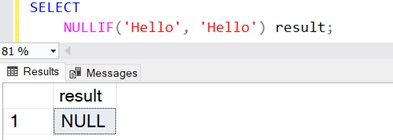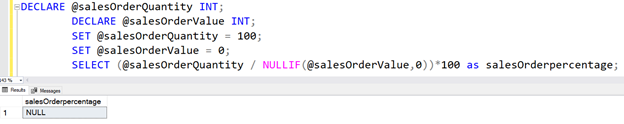top of page

# The SQL NULLIF function

Updated: Jan 18, 2022

The SQL NULLIF is a function that is widely used in SQL. This function looks at two expressions and makes a comparison between them to see if they match. If they match it returns the first expression and if they do not it returns the second expression.

The SQL NULLIF() function takes two arguments and returns the following values.

• If both the arguments are equal, returns NULL

• If both the arguments are not equal, return the first expression.

Syntax of the NULLIF expression:

NULLIF(Expr1, Expr2)

The NULLIF function works equivalent to the following SQL Case statement.

```CASE
WHEN Expr1= Expr2 THEN NULL
ELSE Expr1
END```

Let's understand the SQL NULLIF function using examples.

`SELECT NULLIF(1,2) result; `

It returns the value 1 in the output because both input values are different in the NULLIF function.`SELECT NULLIF(1,1) result`

It returns NULL values because input values are the same.`SELECT NULLIF('Hello',' Hello') result``SELECT NULLIF('Hello',' Hi') result`Use Cases of NULLIF function

Let's understand the use cases of the SQL NULLIF() function.

Avoid divide by zero error in SQL

We get the divide by zero error if the denominator value is zero. It might break your code and give an unwanted error message.

```DECLARE @salesOrderQuantity INT;
DECLARE @salesOrderValue INT;
SET @salesOrderQuantity = 100;
SET @salesOrderValue = 0;
SELECT (@salesOrderQuantity /(@salesOrderValue))*100 as salesOrderpercentage;```Therefore, as shown below, you can modify the SQL query that uses SQLIF() in the denominator to replace a zero value with NULL.

```DECLARE @salesOrderQuantity INT;
DECLARE @salesOrderValue INT;
SET @salesOrderQuantity = 100;
SET @salesOrderValue = 0;
SELECT (@salesOrderQuantity / NULLIF(@salesOrderValue,0))*100 as salesOrderpercentage;```Translate a blank string to NULL

Suppose you have a [DemoTable] with the following data in it. Due to data entry, we did not specify any value for the mobile number column for the second row.

```CREATE TABLE DemoTable
(
ID  INT   PRIMARY KEY ,
[Name] VARCHAR(100) NOT NULL,
MobileNo VARCHAR(20)
);

INSERT INTO DemoTable
(
ID,
[Name],
MobileNo
)
VALUES
(
1,
'A',
'(111)-111-1111'
),
(
2,
'B',
''
),
(
3,
'C',
NULL
);```

If we want to find records that do not have any mobile number, the following query does not show the 2nd record due to an empty string. It lists the column having a NULL value in it.

`SELECT * FROM DemoTable WHERE MobileNo IS NULL`Therefore, we can use the NULLF() function and replace The empty string with a NULL to list both records in the output.

`SELECT * FROM DemoTable WHERE NULLIF(MobileNo,'') IS NULL;`